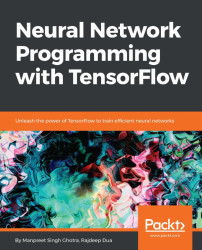•#### Neural Network Programming with Tensorflow#### Overview of this book

If you're aware of the buzz surrounding the terms such as "machine learning," "artificial intelligence," or "deep learning," you might know what neural networks are. Ever wondered how they help in solving complex computational problem efficiently, or how to train efficient neural networks? This book will teach you just that. You will start by getting a quick overview of the popular TensorFlow library and how it is used to train different neural networks. You will get a thorough understanding of the fundamentals and basic math for neural networks and why TensorFlow is a popular choice Then, you will proceed to implement a simple feed forward neural network. Next you will master optimization techniques and algorithms for neural networks using TensorFlow. Further, you will learn to implement some more complex types of neural networks such as convolutional neural networks, recurrent neural networks, and Deep Belief Networks. In the course of the book, you will be working on real-world datasets to get a hands-on understanding of neural network programming. You will also get to train generative models and will learn the applications of autoencoders. By the end of this book, you will have a fair understanding of how you can leverage the power of TensorFlow to train neural networks of varying complexities, without any hassle. While you are learning about various neural network implementations you will learn the underlying mathematics and linear algebra and how they map to the appropriate TensorFlow constructs.
Title PageCreditswww.PacktPub.comCustomer FeedbackPrefaceFree Chapter
Maths for Neural NetworksDeep Feedforward NetworksOptimization for Neural NetworksConvolutional Neural NetworksRecurrent Neural NetworksGenerative ModelsDeep Belief NetworkingAutoencodersResearch in Neural NetworksGetting started with TensorFlow## What is optimization?

The process to find maxima or minima is based on constraints. The choice of optimization algorithm for your deep learning model can mean the difference between good results in minutes, hours, and days.

### Note

Optimization sits at the center of deep learning. Most learning problems reduce to optimization problems. Let's imagine we are solving a problem for some set of data. Using this pre-processed data, we train a model by solving an optimization problem, which optimizes the weights of the model with regards to the chosen loss function and some regularization function.

Hyper parameters of a model play a significant role in the efficient training of a model. Therefore, it is essential to use the different optimization strategies and algorithms to measure appropriate and optimum values of model's hyper parameters, which affect our Model's learning process, and finally the output of a model.